# 6th Grade Geometry Assessment on Finding the Area of Various Objects

Page content

## Assessment Objectives:

The assessment is aligned to the Common Core State Standards for Mathematics – Grade 6 – Geometry

6.G.1 Find the area of right triangles, other triangles, special quadrilaterals, and polygons by composing into rectangles or decomposing into triangles and other shapes, apply these techniques in the context of solving real-world and mathematical problems.

6.G.2 Find the volume of a right rectangular prism with fractional edge lengths by packing it with unit cubes of the appropriate unit fraction edge lengths, and show that the volume is the same as would be found by multiplying the edge lengths of the prism. Apply the formulas to find volumes of right rectangular prisms with fractional edge lengths in the context of solving real-world and mathematical problems.

6.G.3 Draw polygons in the coordinate plane given coordinates for the vertices, use coordinates to find the length of a side joining points with the same first coordinate or the same second coordinate. Apply these techniques in the context of solving real-world and mathematical problems.

6.G.4 Represent three-dimensional figures using nets made up of rectangles and triangles, and use the nets to find the surface area of these figures. Apply these techniques in the context of solving real-world and mathematical problems.

Materials Required: coordinate grid on graph paper, calculator

## Independent Work

**

1. What is the area of each of the right triangle? Show how you found your answer.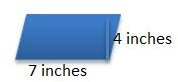2. What is the area of the parallelogram? Show how you found your answer.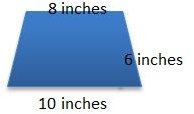3. What is the area of the isosceles trapezoid? Show how you found your answer.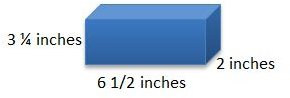4. What is the volume of the right rectangular prism? Show how you found your answer.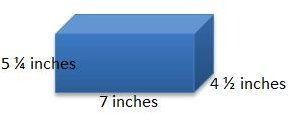5. What is the volume of the right rectangular prism? Show how you found your answer.

On your coordinate grid, draw a rectangle with the vertecies at the following coordinates:

Point A: (-9, 7)
Point B: (2, 7)
Point C: (2, -5)
Point D: (-9, -5)

6. What is the distance between Point A and Point B?

7. What is the distance between Point B and Point C?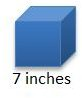8. What is the surface area of the cube?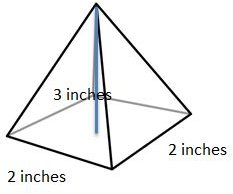9. What is the surface area of the square pyramid?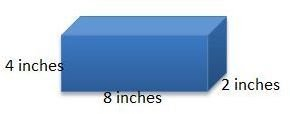10. What is the surface area of the rectangular prism?

1. Area of right triangle is ½ b x h, ½ 12 x 3 = 18 square inches.

2. Area of parallelogram is b x h, 7 x 4 = 28 square inches.

3. Area of trapezoid is area of rectangle + area of 2 traingles. Area of rectangle is 8 x 6 = 48 square inches. Area of each triangle is ½ x 1 x 6 = 3 square inches. Area of trapezoid is 48 + 2 (3) = 54 square inches.

4. Volume of right rectangular prism is 3 ¼ x 6 ½ x 2 = 42 ¼ cubic inches.

5. Volume of right rectangular prism is 4 ½ x 7 x 5 ¼ = 165.375 scubic inches.

6. 11 units

7. 12 units

8. The net for the cube is 6 squares. The surface area for the cube is the sum of the areas of 6 squares, 6 x 7 2 = 6 x 49 = 294 square inches.

9. The net for the square pyramid is 1 square and 4 triangles. The surface area for the square pyramid is the sum of the areas of the sqaure and the 4 triangles, 1 (2 x 2) + 4 x ½ (3 x 2) = 4 + 12 = 16 square inches.

10. The net for the rectangular prism is 4 rectangles with dimensions 8 x 4 and 2 rectangles 4 x 2. The surface area for the rectangular prism is the sum of the areas of the rectangles, 4 (8 x 4) + 2 (4 x 2) = 144 square inches.